Test: Factorisation- 1

# Test: Factorisation- 1

Test Description

## 20 Questions MCQ Test NCERT Mathematics for CAT Preparation | Test: Factorisation- 1

Test: Factorisation- 1 for Class 8 2022 is part of NCERT Mathematics for CAT Preparation preparation. The Test: Factorisation- 1 questions and answers have been prepared according to the Class 8 exam syllabus.The Test: Factorisation- 1 MCQs are made for Class 8 2022 Exam. Find important definitions, questions, notes, meanings, examples, exercises, MCQs and online tests for Test: Factorisation- 1 below.
Solutions of Test: Factorisation- 1 questions in English are available as part of our NCERT Mathematics for CAT Preparation for Class 8 & Test: Factorisation- 1 solutions in Hindi for NCERT Mathematics for CAT Preparation course. Download more important topics, notes, lectures and mock test series for Class 8 Exam by signing up for free. Attempt Test: Factorisation- 1 | 20 questions in 20 minutes | Mock test for Class 8 preparation | Free important questions MCQ to study NCERT Mathematics for CAT Preparation for Class 8 Exam | Download free PDF with solutions
 1 Crore+ students have signed up on EduRev. Have you?
Test: Factorisation- 1 - Question 1

### Divide as directed: 5 (2x + 1) (3x + 5) ÷ (2x + 1)

Detailed Solution for Test: Factorisation- 1 - Question 1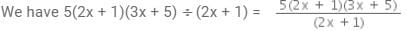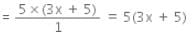∴  5(2x + 1)(3x + 5)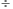(2x + 1) = 5(3x+5)

Test: Factorisation- 1 - Question 2

### Divide as directed: 26xy (x + 5) (y – 4) ÷ 13x (y – 4)

Detailed Solution for Test: Factorisation- 1 - Question 2

26xy(x + 5) (y - 4) / 13x(y - 4)
Cancelling 13x(y-4)
=2y(x+5)

Test: Factorisation- 1 - Question 3

### Find and correct the errors in the following mathematical statements. 4 (x – 5) = 4x – 5

Detailed Solution for Test: Factorisation- 1 - Question 3

By the distributive property a(b-c)=ab- ac
so 4(x-5)=4x-20

Test: Factorisation- 1 - Question 4

Find and correct the errors in the following mathematical statements.

x (3x + 2) = 3x2 + 2

Detailed Solution for Test: Factorisation- 1 - Question 4

x (3x + 2)= 3x2+2x (by distributive property a(b+c)=ab+ac)

Test: Factorisation- 1 - Question 5

Factorise: 10x− 18x+ 14x4

Detailed Solution for Test: Factorisation- 1 - Question 5

10x2 − 18x3 + 14x= 2x(5x− 9x2 + 7x3) = 2x2(5− 9x + 7x2)

Test: Factorisation- 1 - Question 6

Factorise: x2+xy+ 8x+ 8y

Detailed Solution for Test: Factorisation- 1 - Question 6

x^2 + xy + 8x + 8y = x[x + y] + 8[x + y]

= (x + y)(x + 8)

Test: Factorisation- 1 - Question 7

Factorise: 4y−12y + 9

Detailed Solution for Test: Factorisation- 1 - Question 7

We have 4y- 12y+9. comparing the equation with (a-b)2=a2-2ab+b2,gives us a2=(2y)2,2ab=2*3*2y and b2=(3)2.Hence the answer is (2y-3)2.

Test: Factorisation- 1 - Question 8

Solve: 7x2y2z÷ 14xyz

Detailed Solution for Test: Factorisation- 1 - Question 8

7x²y²z²÷14xyz
=(7/14)(x²/x)(y²/y)(z²/z)
=1/2(x)(y)(z)
=1/2xyz.

Test: Factorisation- 1 - Question 9

Find the common factors of 2y, 22xy.

Detailed Solution for Test: Factorisation- 1 - Question 9

Only 2y is common in both the terms

Test: Factorisation- 1 - Question 10

The _______ may be numbers, algebraic variables or algebraic expressions.

Detailed Solution for Test: Factorisation- 1 - Question 10

Factors are numbers we can multiply together to get another number. A constituent or element that brings about certain effects or results, or indicates a specific multiple, number, or quantity.

Test: Factorisation- 1 - Question 11

Factorise : x4 - (y+z)4

Detailed Solution for Test: Factorisation- 1 - Question 11

x4- (y+z)4, applying x- y2=(x-a)(x+a), gives (x2-(y+z)2)(x2+(y+z)2). Again applying the same identity gives (x-(y+z))(x+(y+z))(x2+(y+z)2)

Test: Factorisation- 1 - Question 12

Factorise: x4− (x−z)4

Detailed Solution for Test: Factorisation- 1 - Question 12

x4-(x-z)= (x2-(x-z)2)(x2+(x-z)2) = (x-(x-z))(x+(x-z)(x2+(x-z)2) = z(2x-z)(x2+(x-z)2(Applying a- b= (a+b)(a-b))

Test: Factorisation- 1 - Question 13

Divide the given polynomial by the given monominal: 8 (x3y2z2 + x2y3z2 + x2y2z3) ÷ 4x2y2z2

Detailed Solution for Test: Factorisation- 1 - Question 13

8 (x3y2z2 + x2y3z2 + x2y2z3) ÷ 4x2y2z2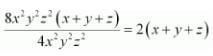Test: Factorisation- 1 - Question 14

Divide the given polynomial by the given monomial: (x3+ 2x2+ 3x) ÷ 2x

Detailed Solution for Test: Factorisation- 1 - Question 14

(x3+2x2+3x)/2x
=x(x2+2x+3)/2x
=(x2+2x+3)/2

Test: Factorisation- 1 - Question 15

Divide as directed: 52pqr (p + q) (q + r) (r + p) ÷104pq (q + r) (r + p)

Detailed Solution for Test: Factorisation- 1 - Question 15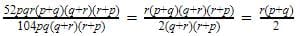Test: Factorisation- 1 - Question 16

Divide as directed: 20 (y+ 4) (y2+ 5y+ 3) ÷ 5(y+ 4)

Detailed Solution for Test: Factorisation- 1 - Question 16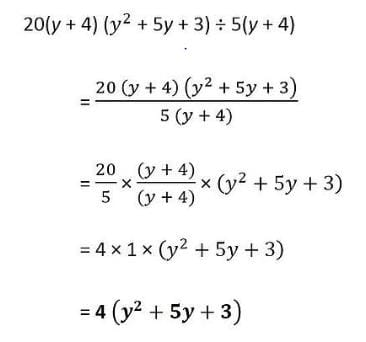Test: Factorisation- 1 - Question 17

Find and correct the errors in the following mathematical statements. 2x + 3y = 5xy

Detailed Solution for Test: Factorisation- 1 - Question 17

LHS = 2x + 3y
RHS = 5xy
LHS ≠ RHS
Correct statement would be
2x + 3y = 2x+3y

Test: Factorisation- 1 - Question 18

Find and correct the errors in the following mathematical statements. x + 2x + 3x = 5x

Detailed Solution for Test: Factorisation- 1 - Question 18

x+2x+3x=6x

Test: Factorisation- 1 - Question 19

Factorise: 12x + 36

Detailed Solution for Test: Factorisation- 1 - Question 19

Let us take 12 as common
and then the factor is 12(x+3) .

Test: Factorisation- 1 - Question 20

Factorise: 15xy – 6x + 5y – 2

Detailed Solution for Test: Factorisation- 1 - Question 20

15xy - 6x + 5y - 2 = 3x[5y-2] + 1[5y-2]
= (5y - 2)(3x + 1)

## NCERT Mathematics for CAT Preparation

276 docs|149 tests
 Use Code STAYHOME200 and get INR 200 additional OFF Use Coupon Code
Information about Test: Factorisation- 1 Page
In this test you can find the Exam questions for Test: Factorisation- 1 solved & explained in the simplest way possible. Besides giving Questions and answers for Test: Factorisation- 1, EduRev gives you an ample number of Online tests for practice

## NCERT Mathematics for CAT Preparation

276 docs|149 tests